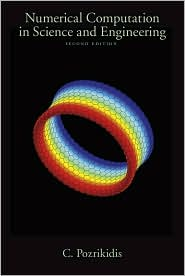Numerical Computation in Science and Engineering

Second Edition, 2008, 1251 Pages, including six appendices

Why this book?

Designed for the non-expert, student, science enthousiast or researcher, this text provides an accessible introduction to numerical computation with applications in science and engineering. It assumes no prior knowledge beyond undergraduate calculus and elementary computer programming. Fundamental and practical issues are discussed in a unified manner with a generous, but not excessive, dose of numerical analysis. Topics are introduced on a need to know basis to concisely illustrate the practical implementation of a variety of algorithms and demystify seemingly esoteric numerical methods. Algorithms that can be explained without too much elaboration and can be implemented within a few dozen lines of computer code are discussed in detail, and computer programs in Fortran, C++, and Matlab are provided. Algorithms whose underlying theories require long, elaborate explanations are discussed at the level of first principles, and references for further information are given. The book uses numerous schematic illustrations to demonstrate concepts and facilitate their understanding by providing readers with a helpful interplay between ideas and visual images. Real-world examples drawn from various branches of science and engineering are presented.

Drawing a direct connection between numerical analysis and numerical computation Numerical Computation in Science and Engineering serves as an ideal text for undergraduate and graduate courses in numerical methods.

Programs

This book is accompanied by a suite of computer programs arranged according to the book chapters.

Errata

1. Page 1:
Line 8: Please replace "in their desks" with "at their desks" (Thanks to Chris Zusi.)
2. Page 84:
Two lines after equation (1.16.8): Please replace "0.0459" with "0.0469" (Thanks to Chris Zusi.)
3. Page 87
Expression (1.1.26): please insert an f at the beginning of the numerator (Thanks to David Schmidt.)
4. Page 186
Line before equation (2.13.6): please modify the equation to read: A u(i) = λ u(i) (Thanks to Bryan Urquhart.)
5. Page 257:
Equations (3.9.39): First equation: Please change "8/5" to "3/4".
Second equation: Please change "3/4" to "8/5" (Thanks to Merlin Ma Wei.)
6. Page 289:
Caption of schematic: Please change to read: "A nonlinear function maps the root of a function, f(x), to the null point."
7. Page 301:
Equation (4.4.30): The three dots should be centered.
8. Page 396:
C++ code at the bottom: Please change "x[i]" to "x[i-1]".
9. Page 444:
Equation (6.13.32): Please insert a comma after "h1"
10. Page 511:
Equations (6.20.18) and (6.20.19): Please change "h2" to "h" near the end of each eqaution.
11. Page 511:
Equation (6.20.20): Please change "h4" to "h2" near the end of this eqaution.
12. Page 690:
Problem 9.1.4, penultimate line: Please change "cB,in" to "cC,in".
13. Page 729:
Equation (9.6.20): Please change "-646" to "+646"
14. Page 806:
Equation (10.2.27): Please put a minus sign (-) after the equal sign (=).
Equation (10.2.30): Please put a minus sign (-) after each of the two equal signs (=).
15. Page 807:
Line after quation (10.2.32): Please replace the sentence "The rest of the eigenvalues are either real or come in pairs of complex conjugates" with the sentence "Using the results of Section 5.2.5, we find that the remaining eigenvalues are all zero."
16. Page 916:
Problem 11.5.3: Please change "11.4.8" to "11.4.7".
17. Page 984:
Please change "0.879 and 5.121" to "1.268 and 4.732", and "1.875 and 4.526" to "2.252 and 4.351".
18. Page 1083:
In the following sentence, please change "and $\d A = \d x \: \d y$ is a differential area in the $xy$ plane." to "and $\d l$ is the arc length along $C$."